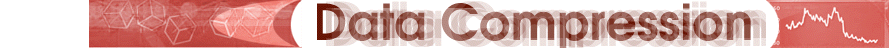The Concept
The Algorithm

- Layer Coding Options
- Hybrid Filter Bank
- Psychoacoustics Model
- Bit Allocation
Layer Coding Options

As the name states, MP3 has three distinct layers for compression. Layer 1 forms the most basic algorithm and the other two layers enhance Layer 1. This section summarizes the main differences between the layers while the following sections delve into details about the stages.

The Layer 1 algorithm codes audio data by grouping together 12 samples from each of the 32 subbands created in the filter bank stage for a total of 384 subbands, as seen in the figure below. Each group of 12 samples gets a bit allocation and scale factor. The bit allocation tells the decoder the number of bits used to represent the sample while the scale factor is a multiplier that sizes the samples.

The Layer 2 algorithm enhances Layer 1 by coding data in larger groups and imposing restrictions on bit allocations for values in higher subbands. The encoder in Layer 2 groups three groups of twelve samples as organized in Layer 1. See the figure below. Additionally, Layer 2 saves bits by representing the bit allocation, scale factor values, and quantized samples (to quantize means to limit the possible values of a magnitude or quantity to a discrete set of values) with more compact code. This allows for more bits to be dedicated to improving audio quality.

Layer 3 is an improvement above the other two layers because it utilizes a transformation known as the Modified Discrete Cosine Transform (MDCT) to represent the frequency of the signal at 32 different frequency bands.Using MPEG compression techniques, data can be reduced to the following percentages while still maintaining CD sound quality:

 25% By Layer 1 16% to 12% By Layer 2 10% to 8% By Layer 3

Next step: Hybrid Filter Bank